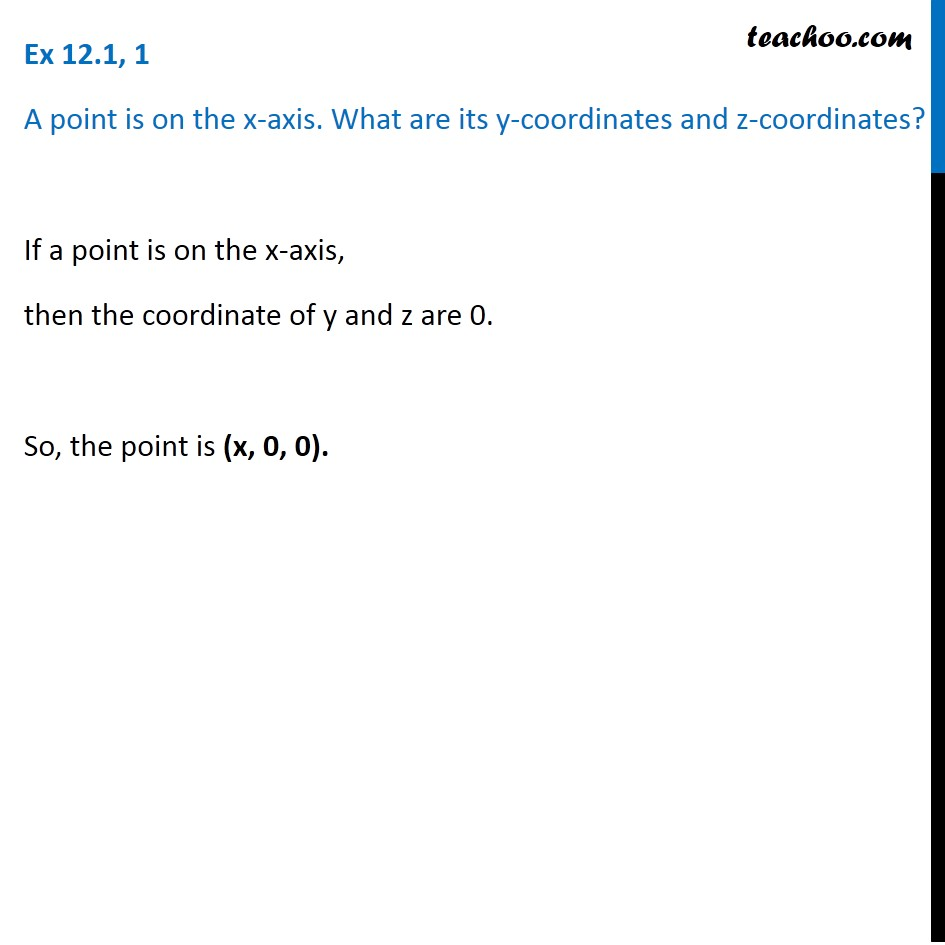Subscribe to our Youtube Channel - https://you.tube/teachoo

1. Chapter 12 Class 11 Introduction to Three Dimensional Geometry
2. Serial order wise
3. Ex 12.1

Transcript

Ex 12.1, 1 A point is on the x-axis. What are its y-coordinates and z-coordinates? If a point is on the x-axis, then the coordinate of y and z are 0. So, the point is (x, 0, 0).

Ex 12.1